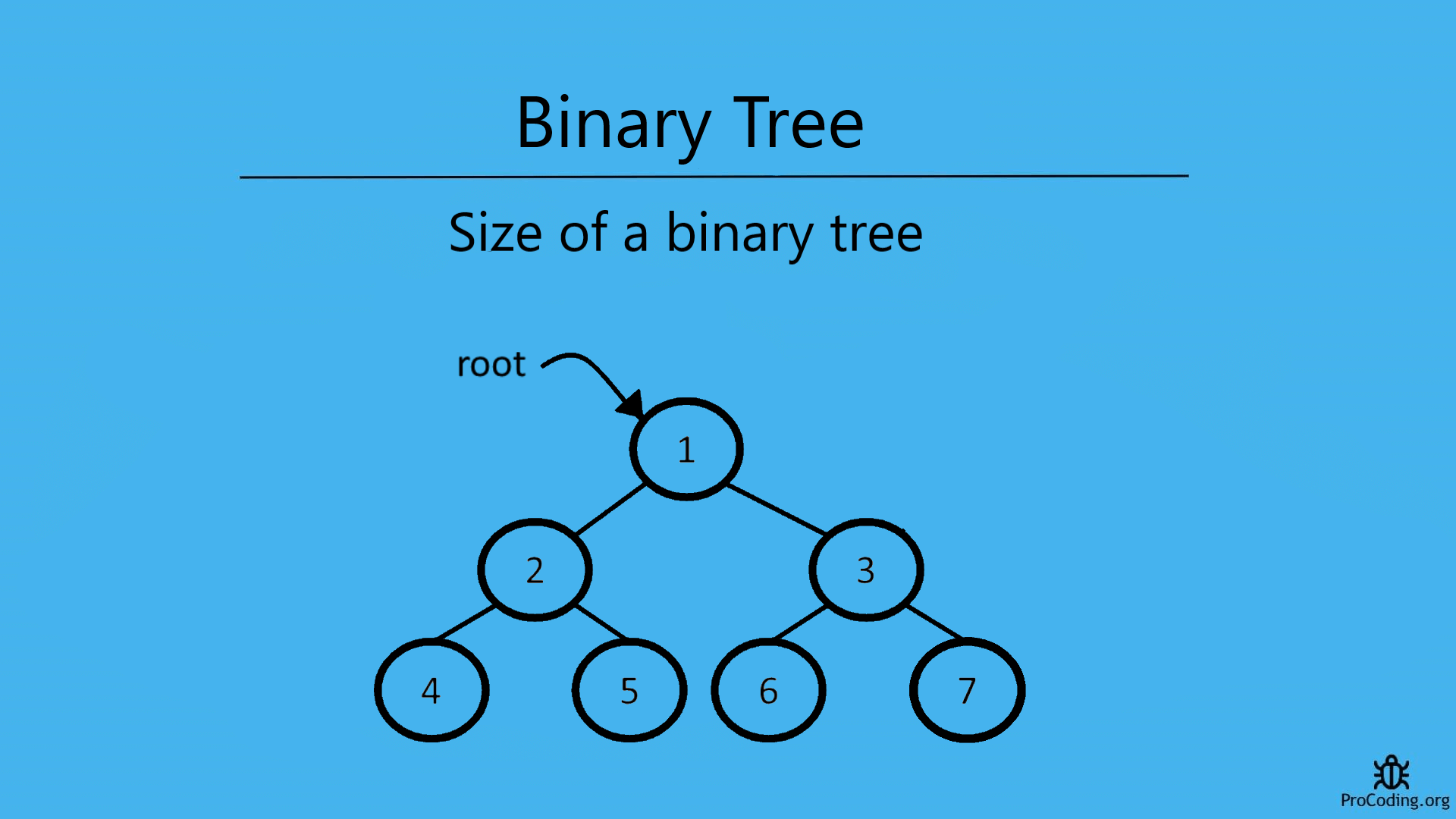# Size of a binary tree

Abhishek Kumar Category: Data StructuresTags: datastructures binary-tree

Find the size of a Binary tree using recursion or iterative solution. For example, a binary tree contains the following elements-
1, 2, 3, 4, 5, 6, 7
SIze of the binary tree will be 7.To find the size of a binary tree we have to traverse each node one by one and count the nodes as long as we are getting nodes in the tree. Same logic can be applied to other trees like the Binary search tree.

We can solve this problem in two ways-

### Recursive solution

For recursive solution we have to check each node one by one recursively as we have done in binary tree recursive traversal. Other types of traversal can also be used.

``````class Node:
def __init__(self, data):
self.left = None
self.data = data
self.right = None

def find_size(root):
if root is None:
return 0
return find_size(root.left) + find_size(root.right) + 1

root = Node(1)
root.left = Node(2)
root.right = Node(3)
root.left.left = Node(4)
root.left.right = Node(5)
root.right.left = Node(6)
root.right.right = Node(7)
print(find_size(root))``````
``````class Node {
constructor(data) {
this.left = null;
this.data = data;
this.right = null;
}
}

function findSize(root) {
if (!root) return 0;
return findSize(root.left) + findSize(root.right) + 1;
}

const root = new Node(1);
root.left = new Node(2);
root.right = new Node(3);
root.left.left = new Node(4);
root.left.right = new Node(5);
root.right.left = new Node(6);
root.right.right = new Node(7);
console.log(findSize(root));``````

Output

`7`

### Iterative solution

For iterative solution, we have to traverse each node in level order and count each node one by one.

``````import queue

class Node:
def __init__(self, data):
self.left = None
self.data = data
self.right = None

def find_size(root):
if root is None:
return 0

q = queue.Queue()
q.put(root)
count = 0
while not q.empty():
count = count + 1
node = q.get()
if node.left:
q.put(node.left)
if node.right:
q.put(node.right)
return count

root = Node(1)
root.left = Node(2)
root.right = Node(3)
root.left.left = Node(4)
root.left.right = Node(5)
root.right.left = Node(6)
root.right.right = Node(7)
print(find_size(root))``````
``````class Node {
constructor(data) {
this.left = null;
this.data = data;
this.right = null;
}
}

function findSize(root) {
if (!root) return 0;

const q = [];
q.push(root);
count = 0;

while (q.length > 0) {
count = count + 1;

let node = q.shift();
if (node.left) {
q.push(node.left);
}
if (node.right) {
q.push(node.right);
}
}
return count;
}

const root = new Node(1);
root.left = new Node(2);
root.right = new Node(3);
root.left.left = new Node(4);
root.left.right = new Node(5);
root.right.left = new Node(6);
root.right.right = new Node(7);
console.log(findSize(root));``````

#### Output

Output

`7`

Time complexity: The time complexity of both of these solution will be O(n) because we are just traversing each element of node one by one.

AUTHOR### Abhishek Kumar

Software engineer | Blogger | Keen learner
Python | Django | JavaScript | React | Next.js | Express.js | C
Passionate about learning and experimenting with new things and open to more opportunities and collaborations.